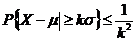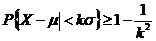# Statistics Assignment Help With Chebycheves Inequality

The role of standard deviation as a parameter to characterize variance is precisely interpreted by means of the well known Chebchev’s inequality.

Theorem:If X is a random variable with mean μ and variance σ2, then for any positive number k, we haveOrProof:

When X is a continuous random variable. By definition